## Errata of Neuro-Fuzzy and Soft Computing

### by J.-S. R. Jang, C.-T. Sun, and E. Mizutani

1.
Page 30: The first equation should be changed from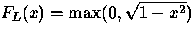to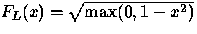.
2.
Page 70, exercise 3: other typoes'' should be max-product''.
3.
Page 70, exercise 5: old and new'' should be young and old''; triangular MFs'' should be Gaussian MFs''. The equations should also be changed to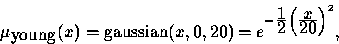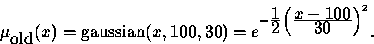4.
Page 70, exercise 6: old and samll'' should be young and old''.
5.
Page 80, line 2: fuzzy mam21 should be changed to ruleview mam21.
6.
Page 113, line 8: (m+1)th should be (k+1)th.
7.
Page 114: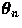in the first line of equation (5.47) should be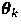. Also the first a in equation (5.49) should be bold.
8.
Page 115: P0 in equation (5.51) should be P0-1.
9.
Page 126: b in Eq. (5.71) and the last equation in the same page should be y. Also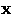in the last equation should be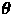.
10.
Page 127: The last equation in Exercise 12 should be11.
Page 133: The second paragraph of Section 6.3: When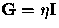, with some positive value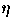and the identity matrix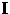'' should be changed to When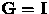(the identity matrix)''.
12.
Page 135, line 17: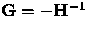should be changed to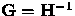.
13.
Page 136: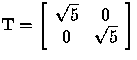should be changed to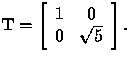14.
Page 138, line 5 from bottom: LM(0.5) when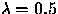and LM(2.0) when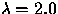'' should be changed to LM(1) when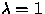and LM(4) when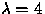''. Similarly, LM(0.5) and LM(2.0) in the caption and plot (c) of Figure 6.6 should be changed to LM(1) and LM(4), respectively.
15.
Page 145, line 9 from bottom: one-third'' should be changed to two-thirds''.
16.
Page 153: gkT'' in the denominator of the last equation should be changed to gk''.
17.
Page 161: The first J in the last equation should be JT.
18.
Page 215: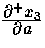in Equation (8.18) should be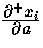.
19.
Pages 217 and 218: The weight between node 4 and 6 of Figure 8.16 and 8.17 should be w64.
20.
Page 311, line 5 from bottom: steepest-descent'' should be steepest-ascent''; minimizes'' should be maximizes''.
21.
Page 313, line 9 from bottom: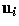should be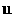.
22.
Page 314: pi in the second and third equations should be changed to qi.
23.
Page 314, line 10 from bottom: the second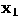should be changed to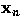.
24.
Page 329, line 1: descent'' should be ascent''; minimizing'' should be maximizing''.
25.
Page 373, line 3 from bottom: illustrating the simplified contours'' should be deleted.
26.
Page 374: C2i+j in the first equation should be changed to C4(i-1)+j.

27.
Page 426: the summation subscripts in Eq. (15.6) and (15.7) should be j=1 instead of j only.

If you find any typos/mistakes, please let us know via email at jang@cs.nthu.edu.tw. Thanks!
The Book's Home Page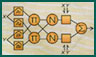, or Roger's Home Page